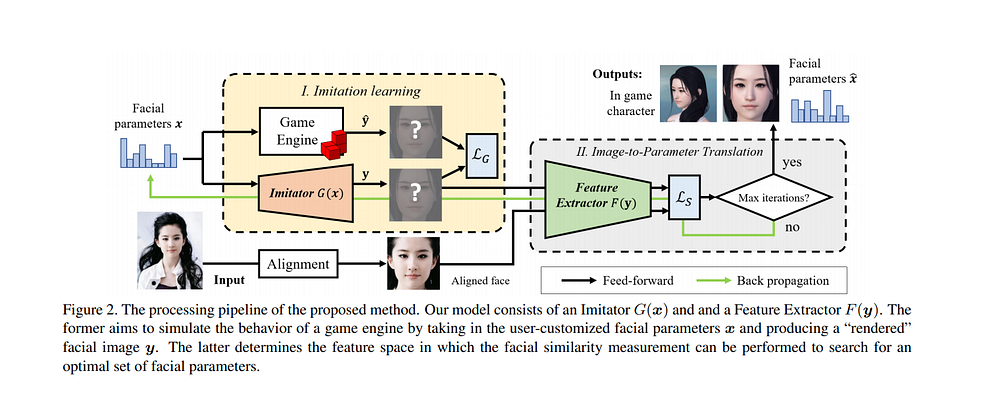Source: Deep Learning on MediumIn Role-Playing Games(RPGs), the character customization system is an important part. Players are allowed to edit the facial parameters of in-game characters. Netease Fuxi AI Lab has released a paper named Face-to-Parameter Translation for Game Character Auto-Creation which proposed an end-to-end approach for face-to-parameter translation and game character auto-creation method. Today, let’s focus on how to reimplement this method.

# Overview

The whole processing pipeline is shown above(Fig.1). The Imitator aims to imitate the behavior of a game engine by taking in user-customized facial parameters and producing a facial image. The Feature Extractor focuses on extracting two kinds of features, 256-d facial embeddings and the facial semantic features on the real-world images and the rendered game characters. The final part is the optimization part. The gradient descent method is used to solve the optimization problem. The following parts are the details on each component’s implement.

# pre-requisites

Unity-3d (≥ 2018.3.14 required)

Tensorflow (≥ 1.12 required)

# Imitator

Before training the Imitator, we used Unity-3d to design a male character that has 216 facial parameters. Some rendered faces are shown in Fig. 2. We randomly generated 20,000 individual faces with their corresponding facial customization parameters for training.

The Imitator is based on DC-GAN’s generator. The whole structure of the network is shown below. Unlike the training process of the GANs, the imitator is fully supervised. The input is the facial parameters and the output is the front view of the game character. So the imitator is similar to the decoder of an Auto-encoder network(Fig.4).

`model = Sequential()model.add(Dense(256 * 4 * 4, activation="relu", input_dim=self.latent_dim))model.add(Reshape((4, 4, 256)))model.add(UpSampling2D())model.add(Conv2D(256, kernel_size=4, padding="same"))model.add(BatchNormalization(momentum=0.8))model.add(Activation("relu"))model.add(UpSampling2D())model.add(Conv2D(256, kernel_size=4, padding="same"))model.add(BatchNormalization(momentum=0.8))model.add(Activation("relu"))model.add(UpSampling2D())model.add(Conv2D(128, kernel_size=4, padding="same"))model.add(BatchNormalization(momentum=0.8))model.add(Activation("relu"))model.add(UpSampling2D())model.add(Conv2D(64, kernel_size=4, padding="same"))model.add(BatchNormalization(momentum=0.8))model.add(Activation("relu"))model.add(UpSampling2D())model.add(Conv2D(32, kernel_size=4, padding="same"))model.add(BatchNormalization(momentum=0.8))model.add(Activation("relu"))model.add(UpSampling2D())model.add(Conv2D(self.channels, kernel_size=4, padding="same")) model.add(Activation("tanh"))`

We used the SGD optimizer with the batch_size = 16 and momentum = 0.9. The learning rate is set to 0.01 and the loss function is Mean_Absolute_Error.

`optimizer = SGD(0.01, 0.9)self.generator.compile(loss='mae', optimizer=optimizer)`

As the paper says, the training stops after 500 training epochs and the learning rate decay is set to 10% per 50 epochs. After training, the performance of the Imitator is very good. Some details of the generated images(Fig. 5) are very similar to images that the game engine produced.